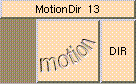MOTIONDIRTHE BOX'S MAIN HELP: This returns the vector describing the direction of the motion. It is used in calculating motion blur. If there is no motion a null vector is returned. DIR (output): This returns the vector describing the direction of the motion. It is used in calculating motion blur. If there is no motion a null vector is returned.

<-- BACK TO Math